# PCA Data Representn Cont PCA Data Representn Cont

• Slides: 76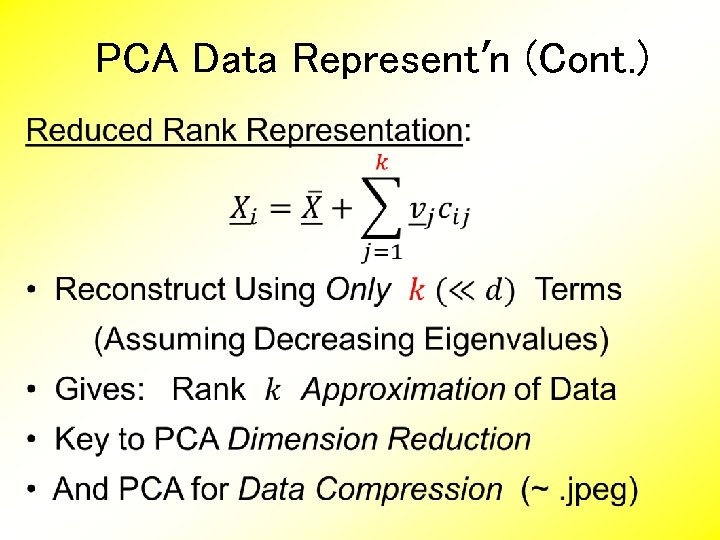PCA Data Represent’n (Cont. )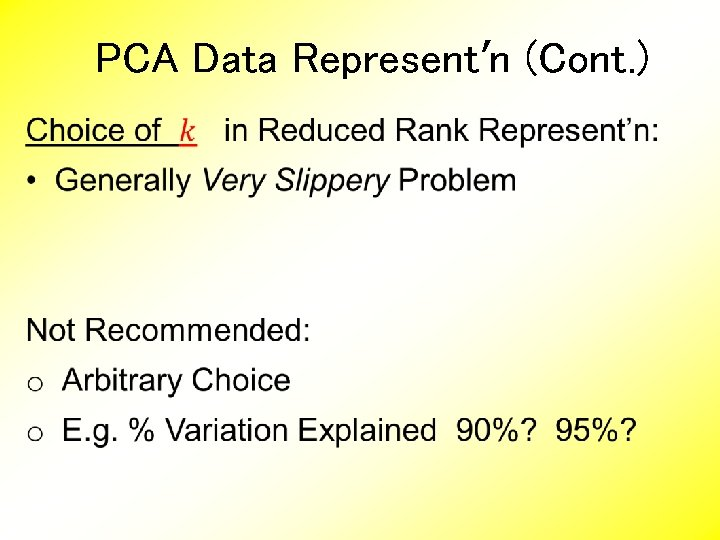PCA Data Represent’n (Cont. )PCA Simulation Idea: given • Mean Vector • Eigenvectors • Eigenvalues Simulate data from Corresponding Normal Distribution Approach: Invert PCA Data Represent’n where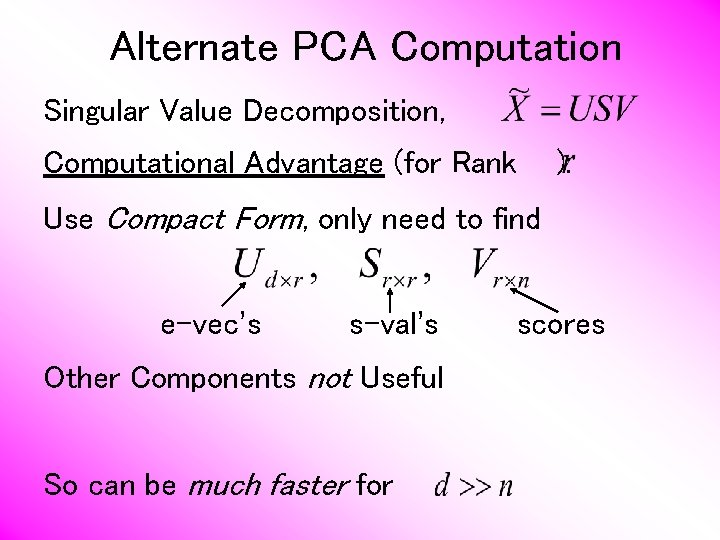Alternate PCA Computation Singular Value Decomposition, Computational Advantage (for Rank ): Use Compact Form, only need to find e-vec’s s-val’s Other Components not Useful So can be much faster for scores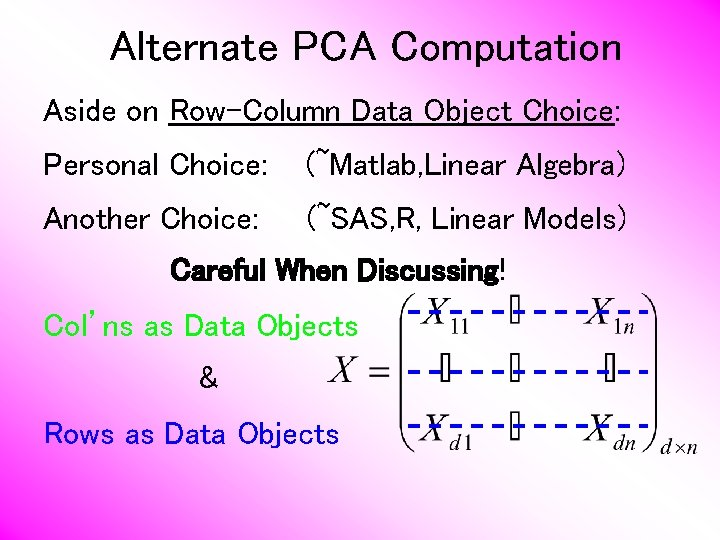Alternate PCA Computation Aside on Row-Column Data Object Choice: Personal Choice: (~Matlab, Linear Algebra) Another Choice: (~SAS, R, Linear Models) Careful When Discussing! Col’ns as Data Objects & Rows as Data Objects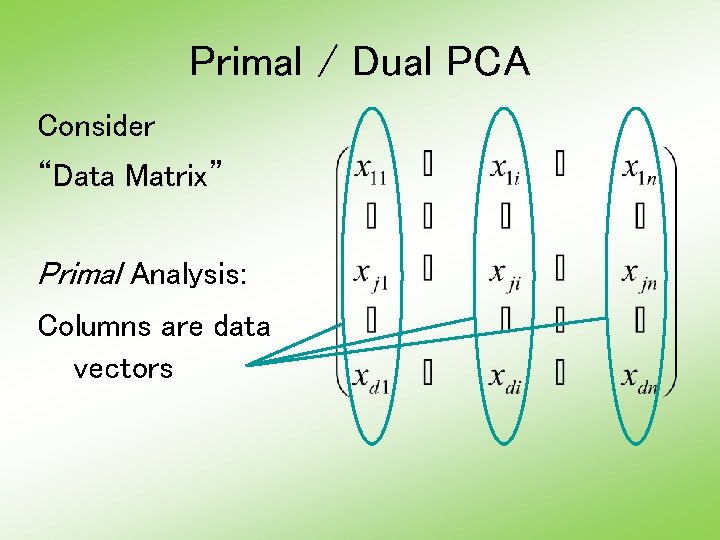Primal / Dual PCA Consider “Data Matrix” Primal Analysis: Columns are data vectors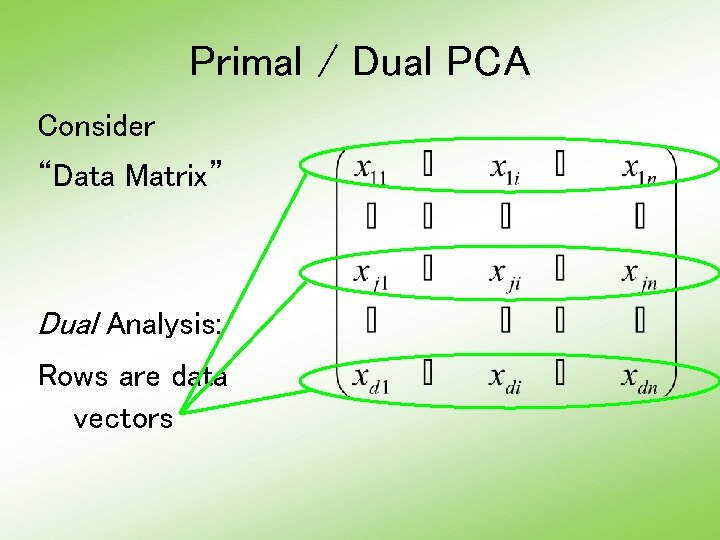Primal / Dual PCA Consider “Data Matrix” Dual Analysis: Rows are data vectorsDemography Data Recall Primal - Raw Data Rainbow Color Scheme Allowed Good Interpretation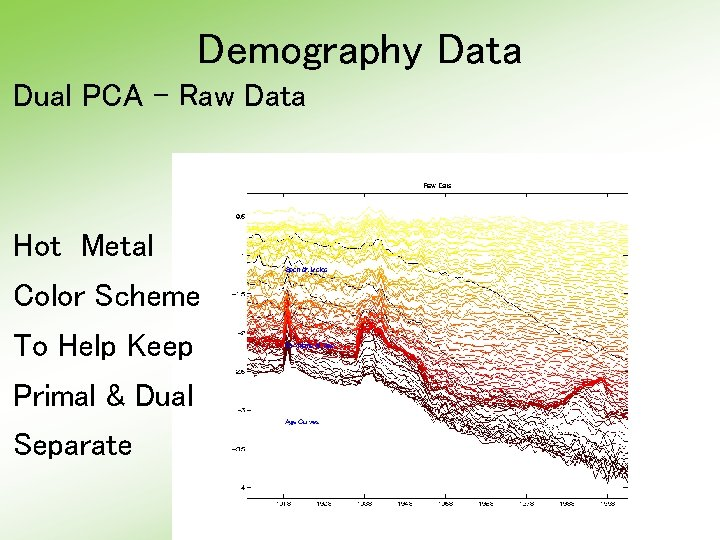Demography Data Dual PCA - Raw Data Hot Metal Color Scheme To Help Keep Primal & Dual Separate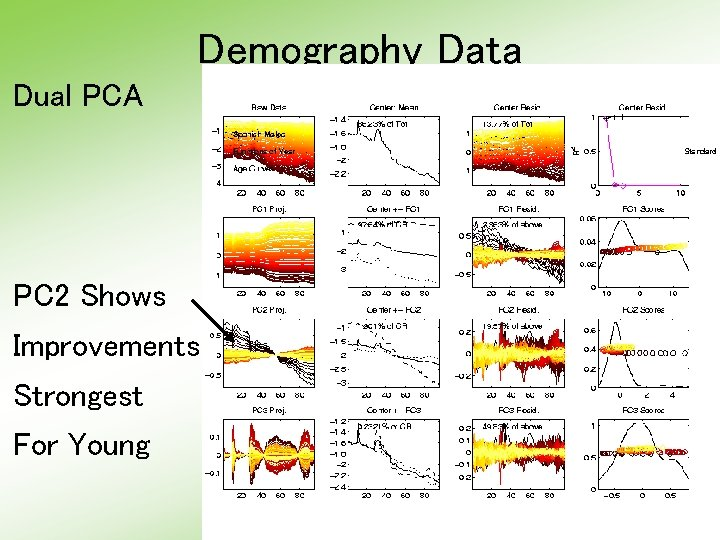Demography Data Dual PCA PC 2 Shows Improvements Strongest For YoungDemography Data Dual PCA Scores Note PC 2 & PC 1 Together Show Mortality vs. Age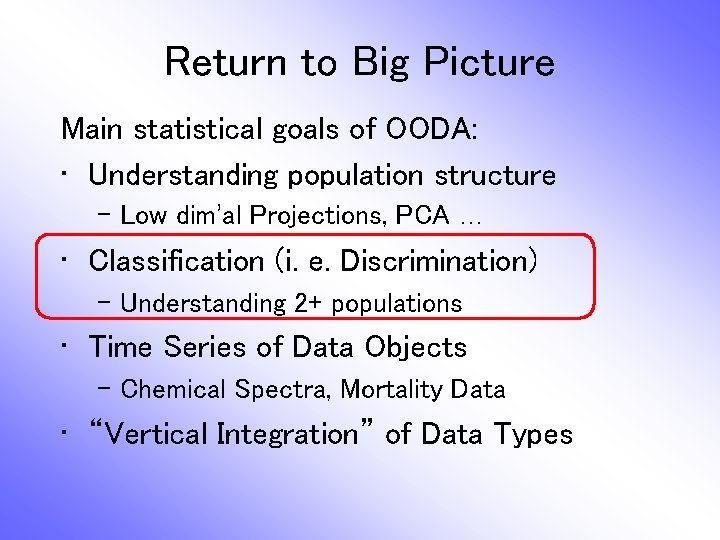Return to Big Picture Main statistical goals of OODA: • Understanding population structure – Low dim’al Projections, PCA … • Classification (i. e. Discrimination) – Understanding 2+ populations • Time Series of Data Objects – Chemical Spectra, Mortality Data • “Vertical Integration” of Data TypesClassification - Discrimination Background: Two Class (Binary) version: Using “training data” from Class +1 and Class -1 Develop a “rule” for assigning new data to a Class Canonical Example: Disease Diagnosis • New Patients are “Healthy” or “Ill” • Determine based on measurements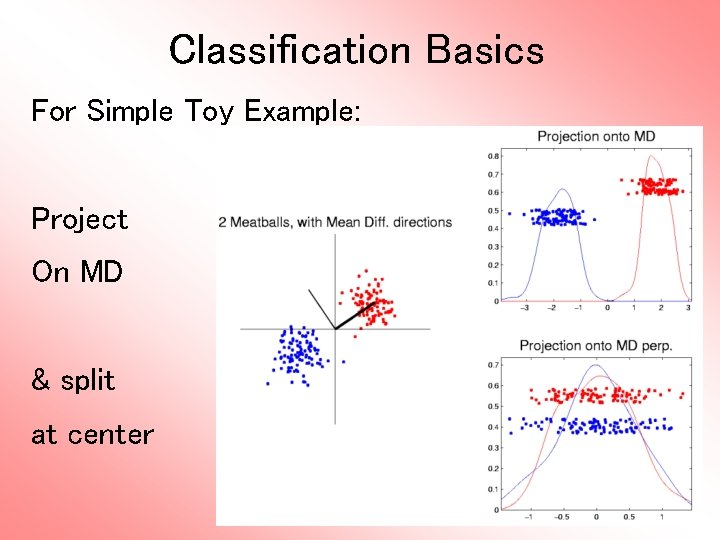Classification Basics For Simple Toy Example: Project On MD & split at center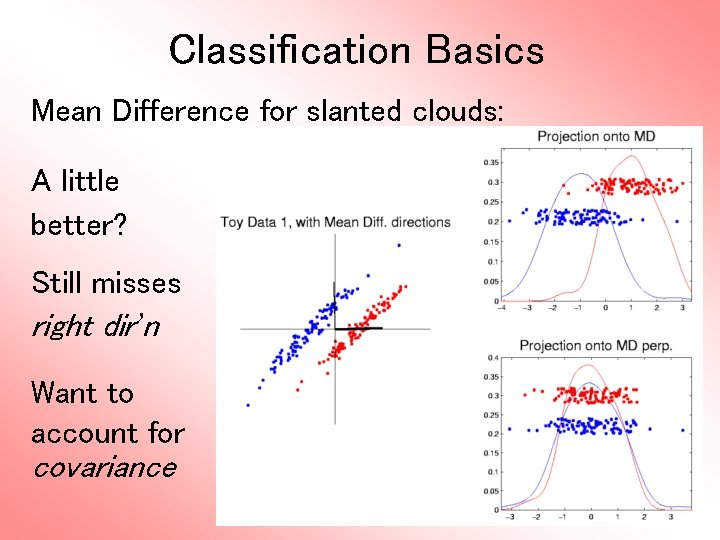Classification Basics Mean Difference for slanted clouds: A little better? Still misses right dir’n Want to account for covariance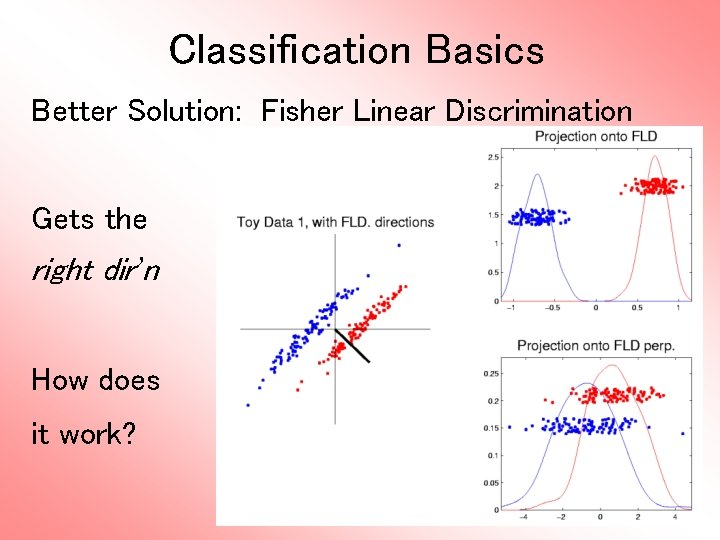Classification Basics Better Solution: Fisher Linear Discrimination Gets the right dir’n How does it work?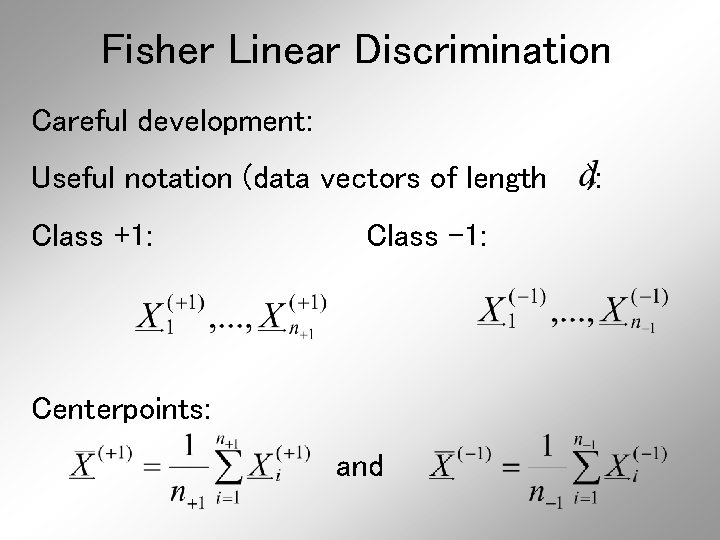Fisher Linear Discrimination Careful development: Useful notation (data vectors of length Class +1: Class -1: Centerpoints: and ):Fisher Linear Discrimination Covariances, for (outer products) Based on centered, normalized data matrices: Note: use “MLE” version of estimated covariance matrices, for simpler notation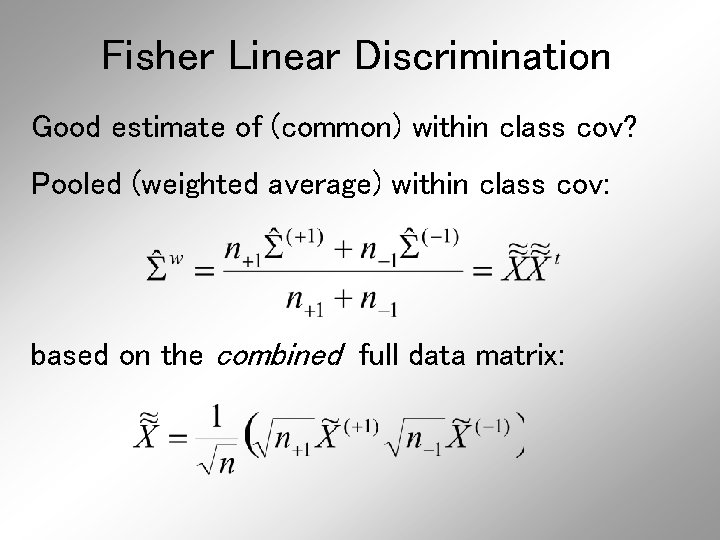Fisher Linear Discrimination Good estimate of (common) within class cov? Pooled (weighted average) within class cov: based on the combined full data matrix: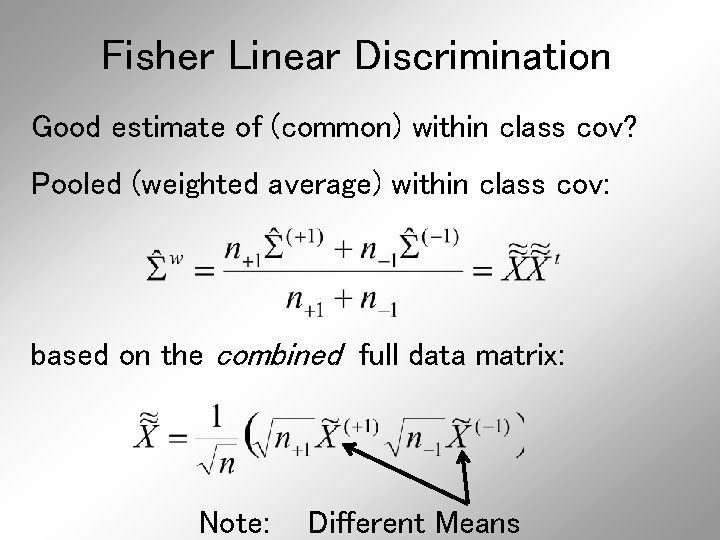Fisher Linear Discrimination Good estimate of (common) within class cov? Pooled (weighted average) within class cov: based on the combined full data matrix: Note: Different MeansFisher Linear Discrimination Note: is similar to from before I. e. covariance matrix ignoring class labels Important Difference: Class by Class Centering Will be important later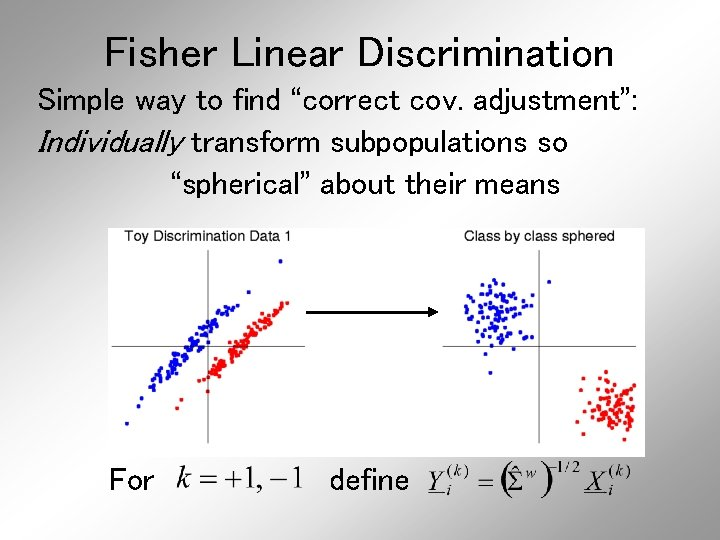Fisher Linear Discrimination Simple way to find “correct cov. adjustment”: Individually transform subpopulations so “spherical” about their means For define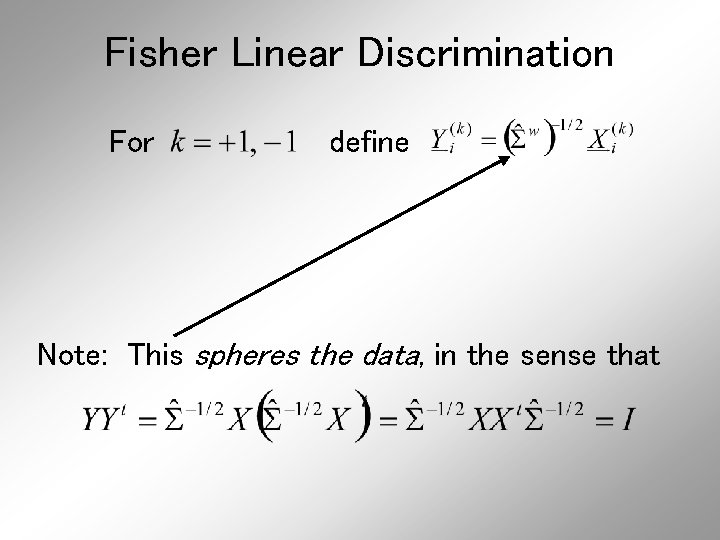Fisher Linear Discrimination For define Note: This spheres the data, in the sense thatFisher Linear Discrimination Then: In Transformed Space, Best separating hyperplane is Perpendicular bisector of line between means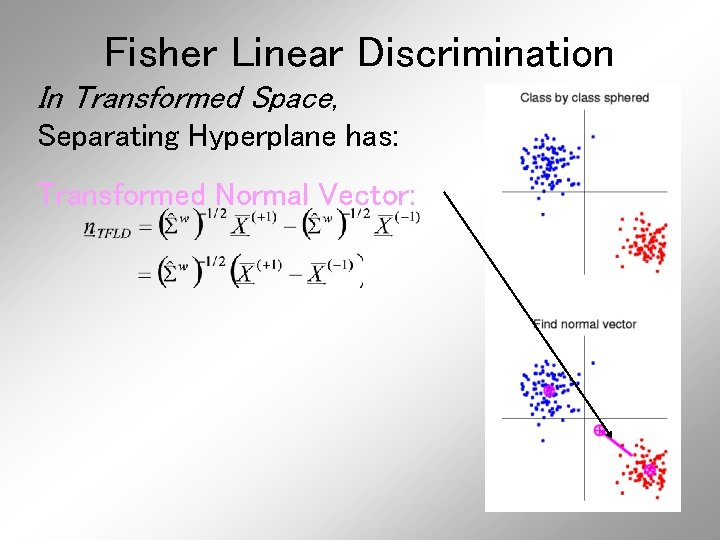Fisher Linear Discrimination In Transformed Space, Separating Hyperplane has: Transformed Normal Vector: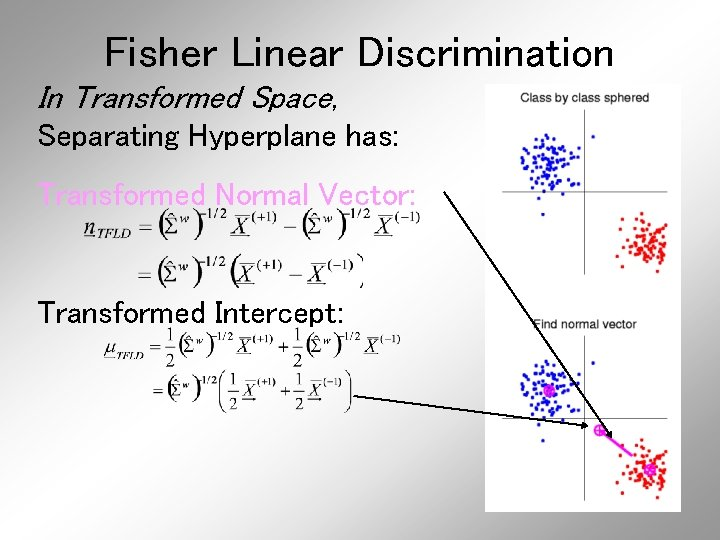Fisher Linear Discrimination In Transformed Space, Separating Hyperplane has: Transformed Normal Vector: Transformed Intercept:Fisher Linear Discrimination In Transformed Space, Separating Hyperplane has: Transformed Normal Vector: Transformed Intercept: Sep. Hyperp. has Equation: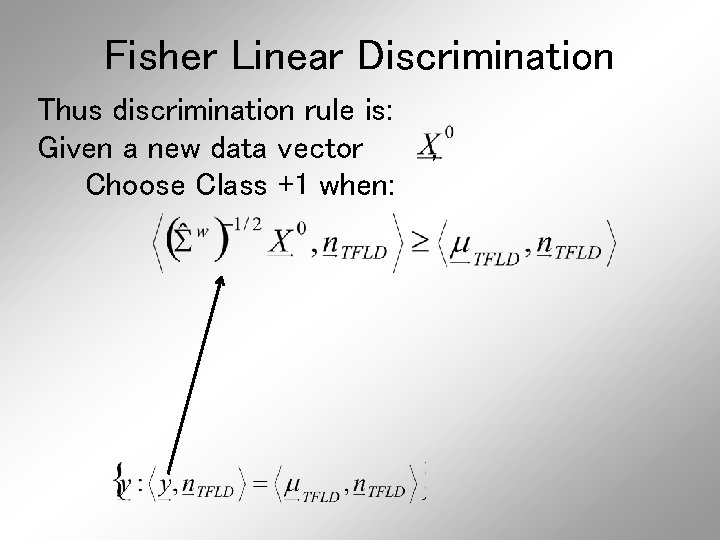Fisher Linear Discrimination Thus discrimination rule is: Given a new data vector Choose Class +1 when: ,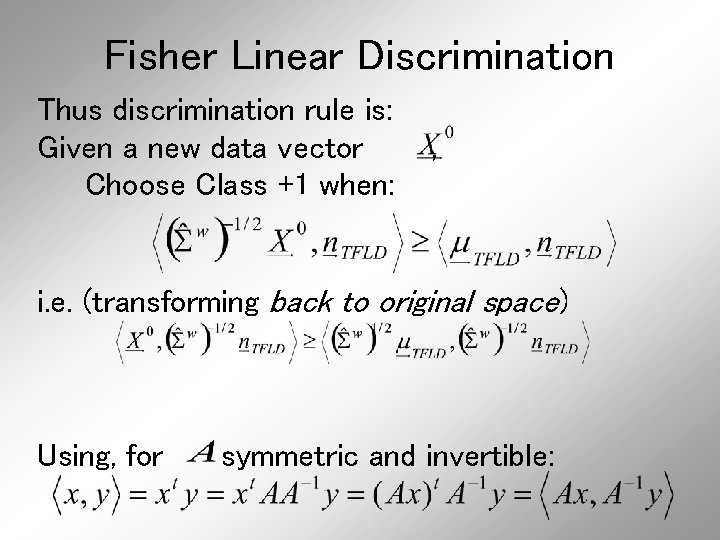Fisher Linear Discrimination Thus discrimination rule is: Given a new data vector Choose Class +1 when: , i. e. (transforming back to original space) Using, for symmetric and invertible: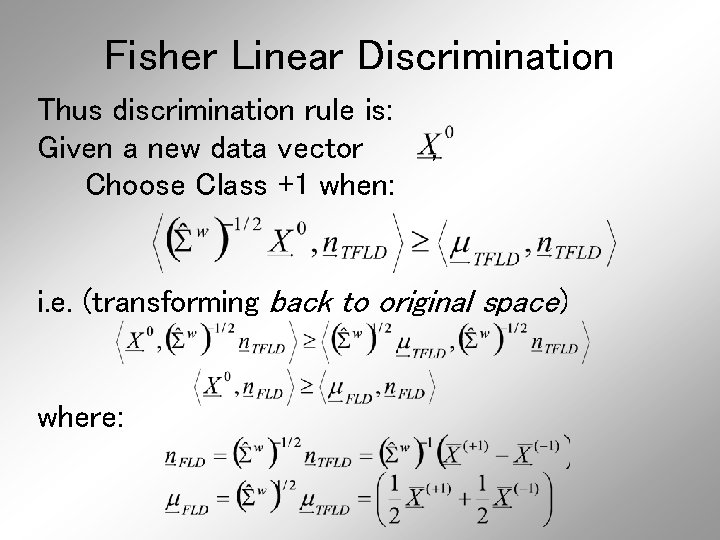Fisher Linear Discrimination Thus discrimination rule is: Given a new data vector Choose Class +1 when: , i. e. (transforming back to original space) where: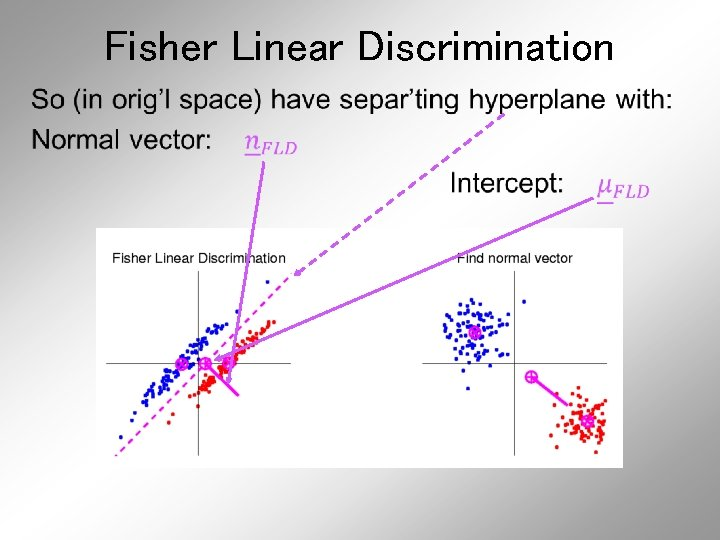Fisher Linear Discrimination •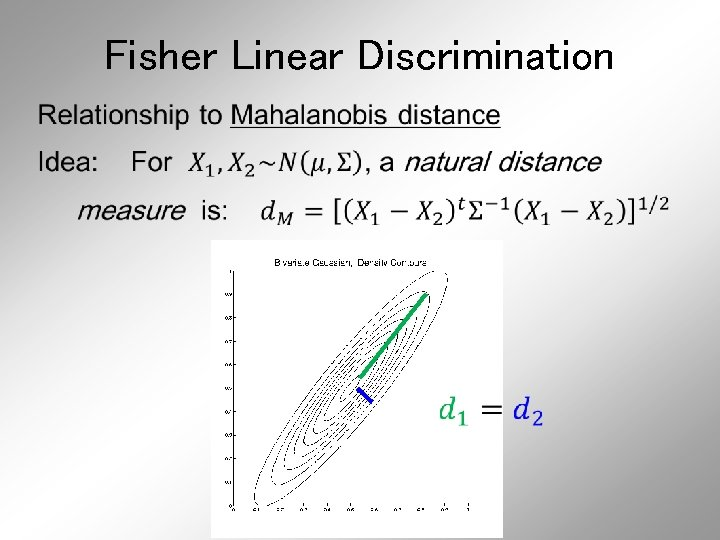Fisher Linear Discrimination •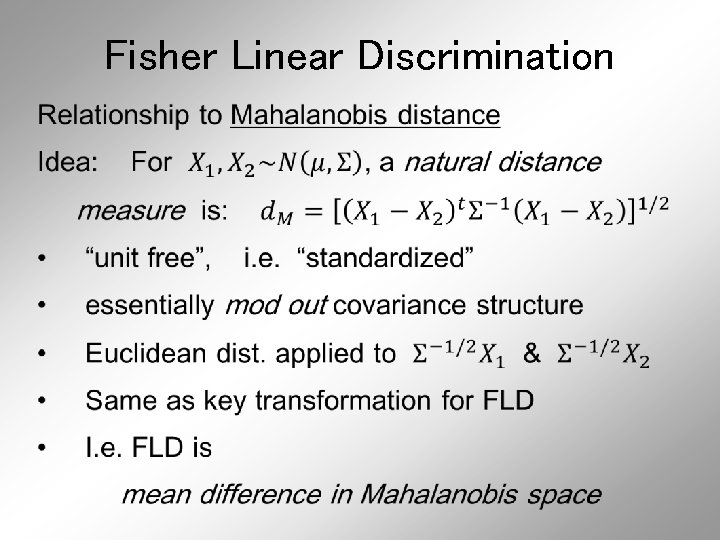Fisher Linear Discrimination •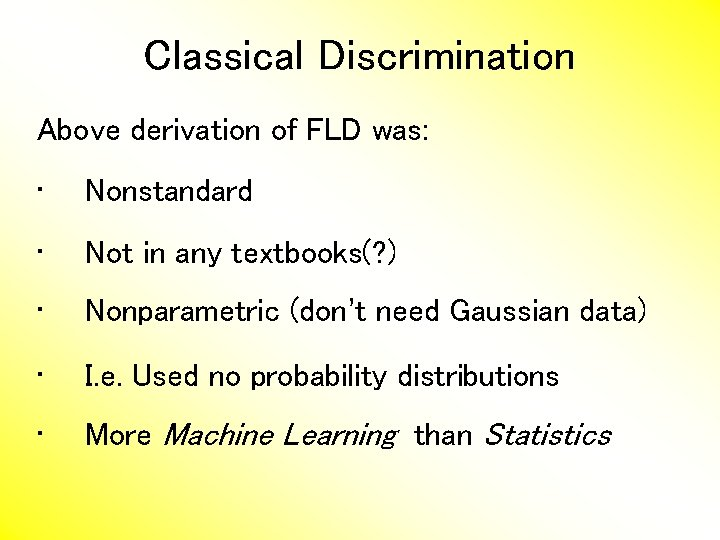Classical Discrimination Above derivation of FLD was: • Nonstandard • Not in any textbooks(? ) • Nonparametric (don’t need Gaussian data) • I. e. Used no probability distributions • More Machine Learning than StatisticsClassical Discrimination FLD Likelihood View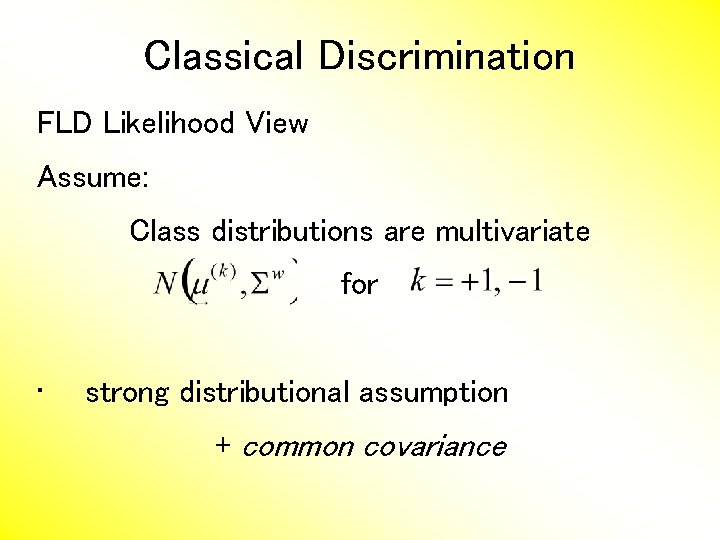Classical Discrimination FLD Likelihood View Assume: Class distributions are multivariate for • strong distributional assumption + common covarianceClassical Discrimination FLD Likelihood View (cont. ) At a location , the likelihood ratio, for choosing between Class +1 and Class -1, is: where is the Gaussian density with covariance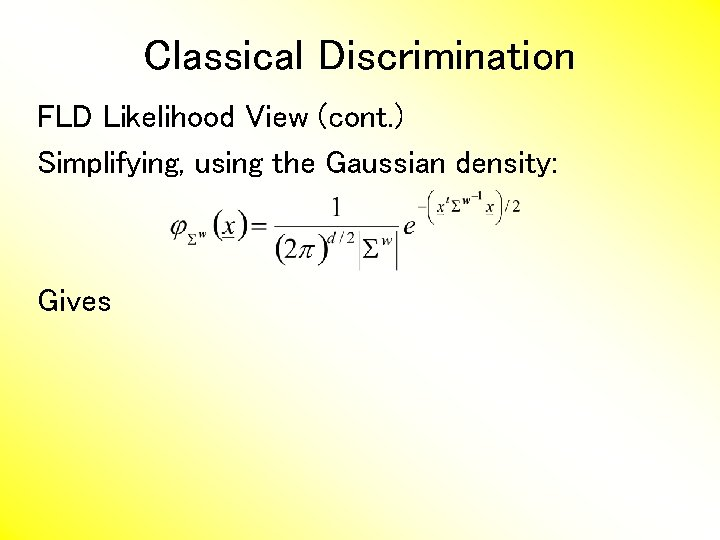Classical Discrimination FLD Likelihood View (cont. ) Simplifying, using the Gaussian density: Gives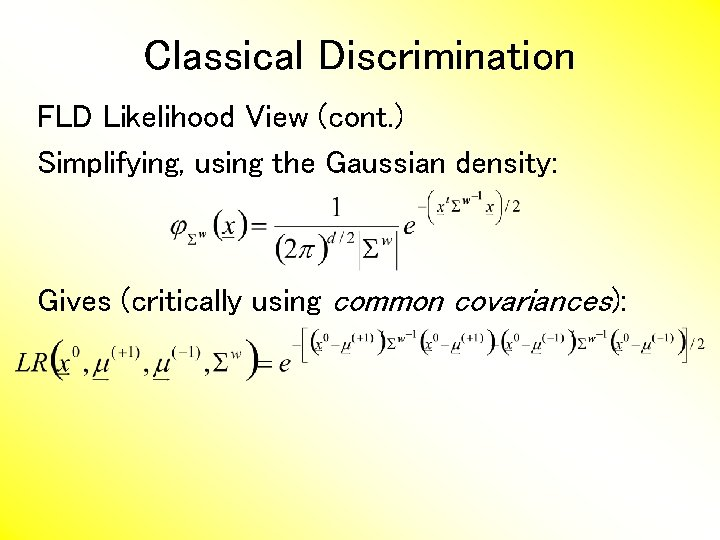Classical Discrimination FLD Likelihood View (cont. ) Simplifying, using the Gaussian density: Gives (critically using common covariances):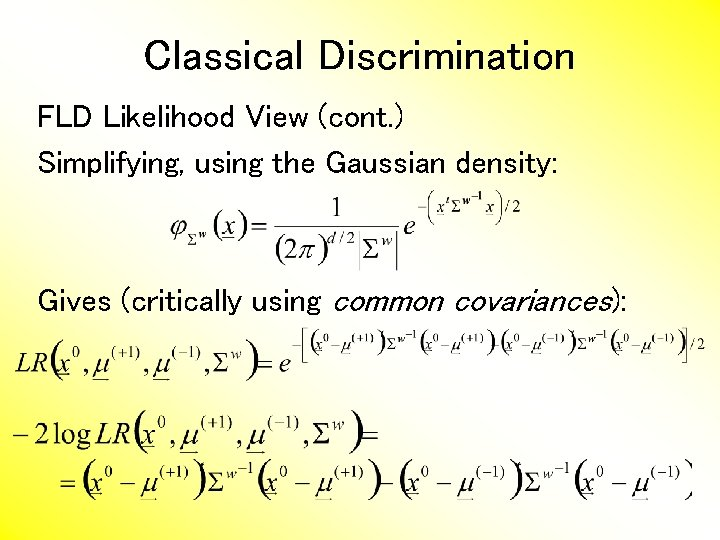Classical Discrimination FLD Likelihood View (cont. ) Simplifying, using the Gaussian density: Gives (critically using common covariances):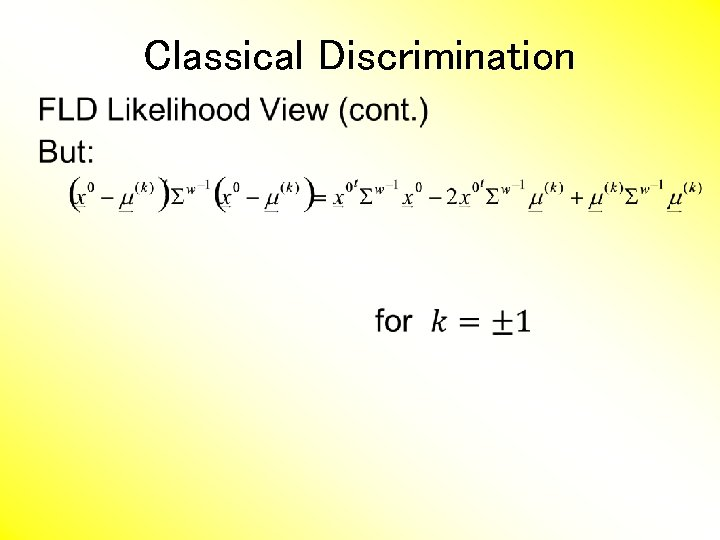Classical Discrimination •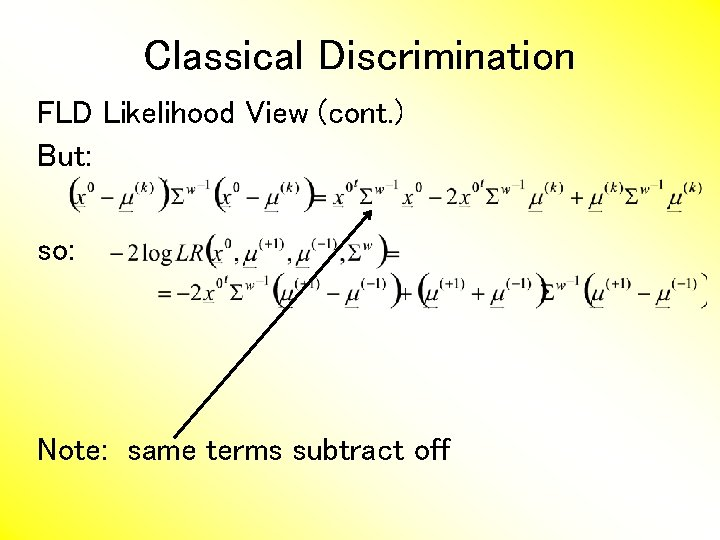Classical Discrimination FLD Likelihood View (cont. ) But: so: Note: same terms subtract off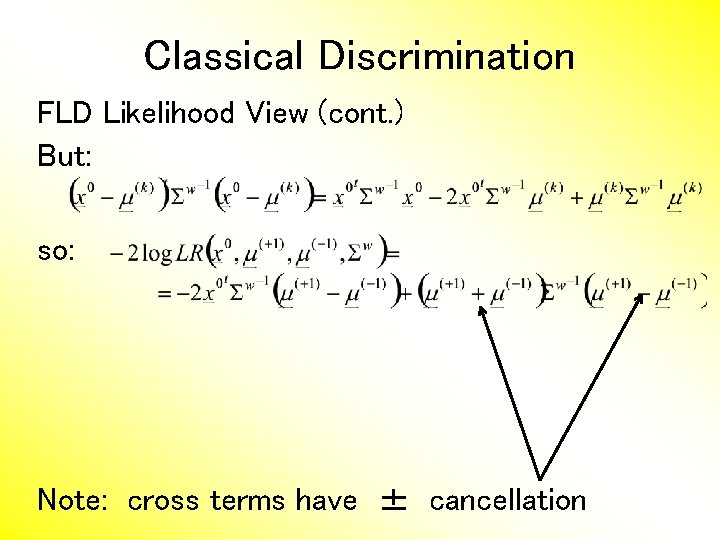Classical Discrimination FLD Likelihood View (cont. ) But: so: Note: cross terms have ± cancellationClassical Discrimination FLD Likelihood View (cont. ) But: so: Thus when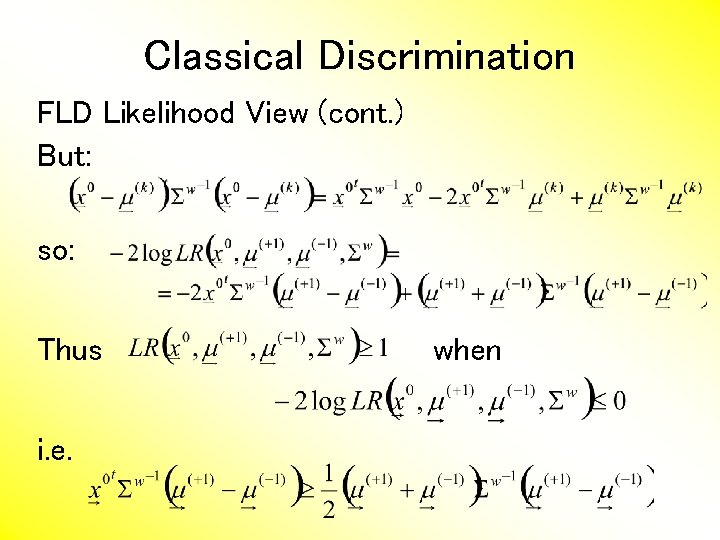Classical Discrimination FLD Likelihood View (cont. ) But: so: Thus i. e. when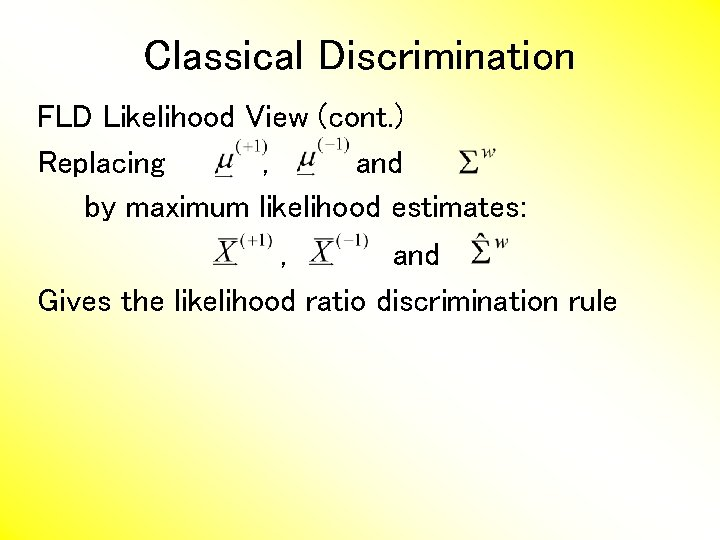Classical Discrimination FLD Likelihood View (cont. ) Replacing , and by maximum likelihood estimates: , and Gives the likelihood ratio discrimination rule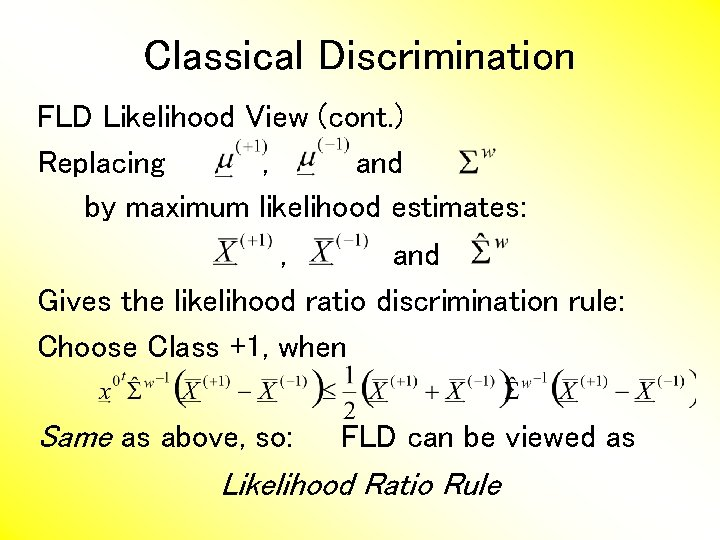Classical Discrimination FLD Likelihood View (cont. ) Replacing , and by maximum likelihood estimates: , and Gives the likelihood ratio discrimination rule: Choose Class +1, when Same as above, so: FLD can be viewed as Likelihood Ratio Rule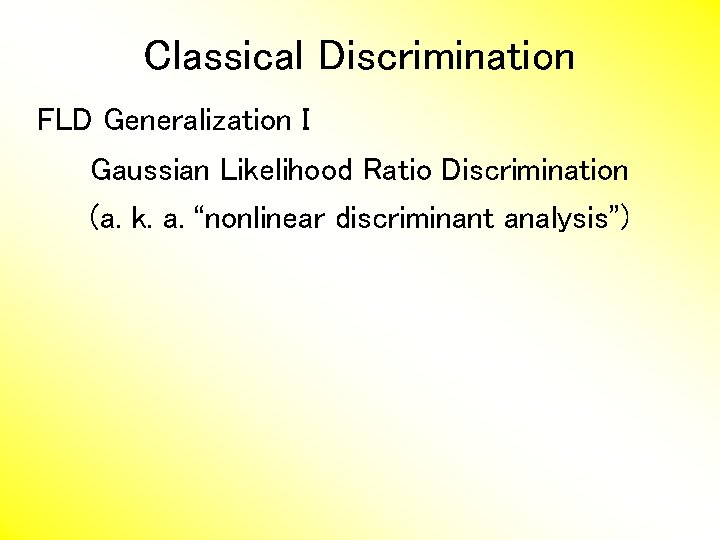Classical Discrimination FLD Generalization I Gaussian Likelihood Ratio Discrimination (a. k. a. “nonlinear discriminant analysis”)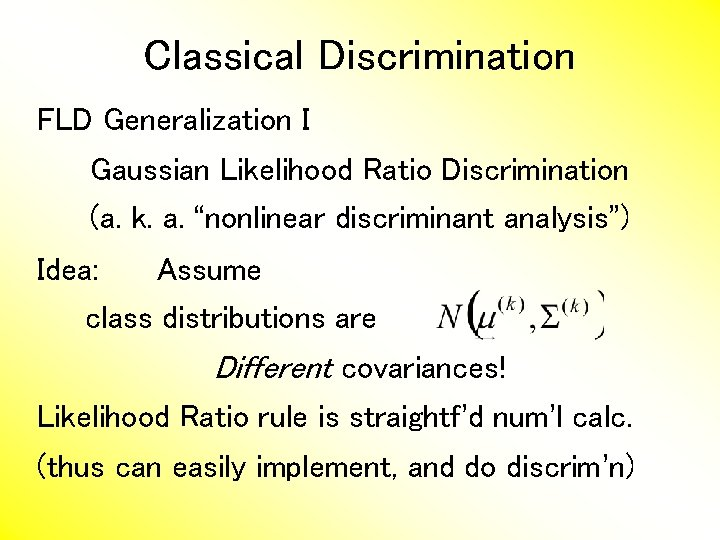Classical Discrimination FLD Generalization I Gaussian Likelihood Ratio Discrimination (a. k. a. “nonlinear discriminant analysis”) Idea: Assume class distributions are Different covariances! Likelihood Ratio rule is straightf’d num’l calc. (thus can easily implement, and do discrim’n)Classical Discrimination Gaussian Likelihood Ratio Discrim’n (cont. ) No longer have separ’g hyperplane repr’n (instead regions determined by quadratics) (fairly complicated case-wise calculations) Graphical display: for each point, color as: Yellow if assigned to Class +1 Cyan if assigned to Class -1 (intensity is strength of assignment)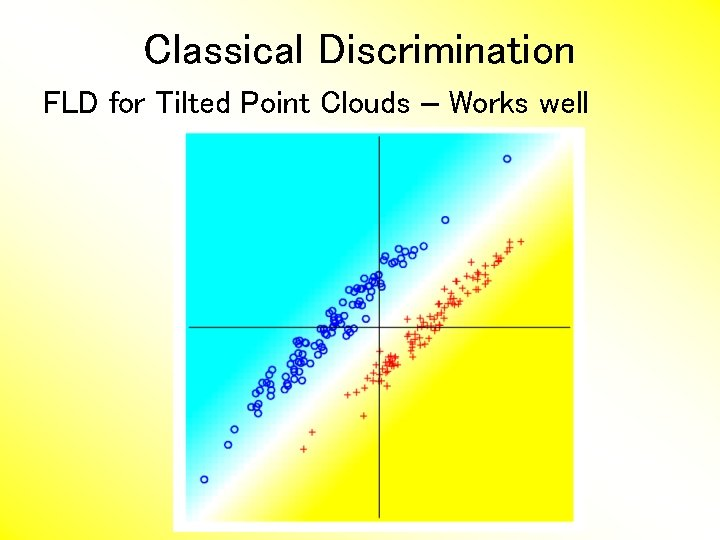Classical Discrimination FLD for Tilted Point Clouds – Works wellClassical Discrimination GLR for Tilted Point Clouds – Works well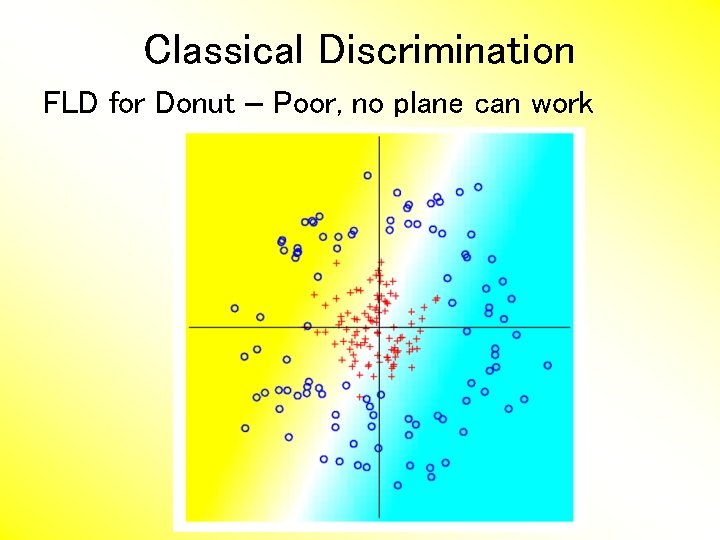Classical Discrimination FLD for Donut – Poor, no plane can work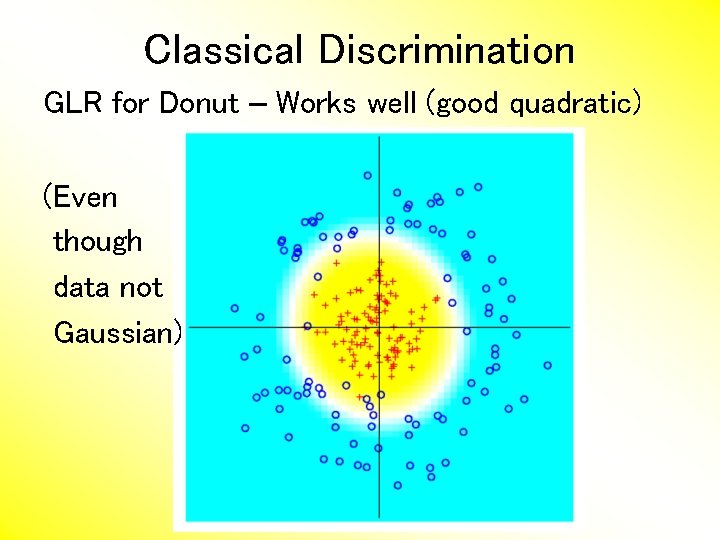Classical Discrimination GLR for Donut – Works well (good quadratic) (Even though data not Gaussian)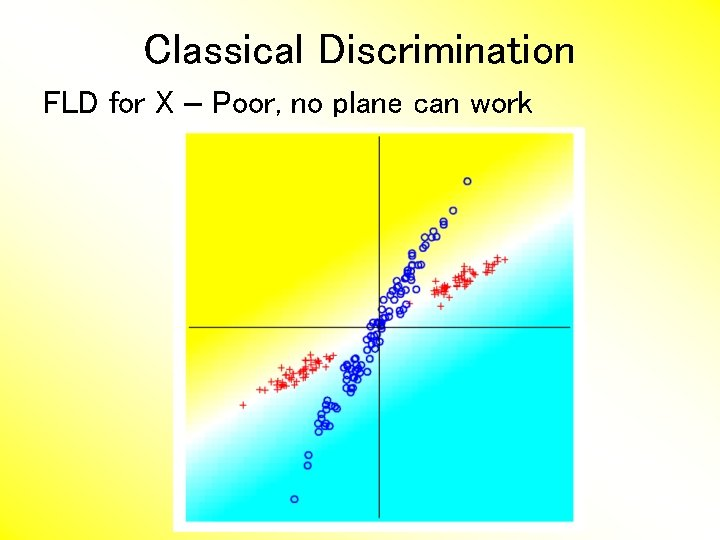Classical Discrimination FLD for X – Poor, no plane can work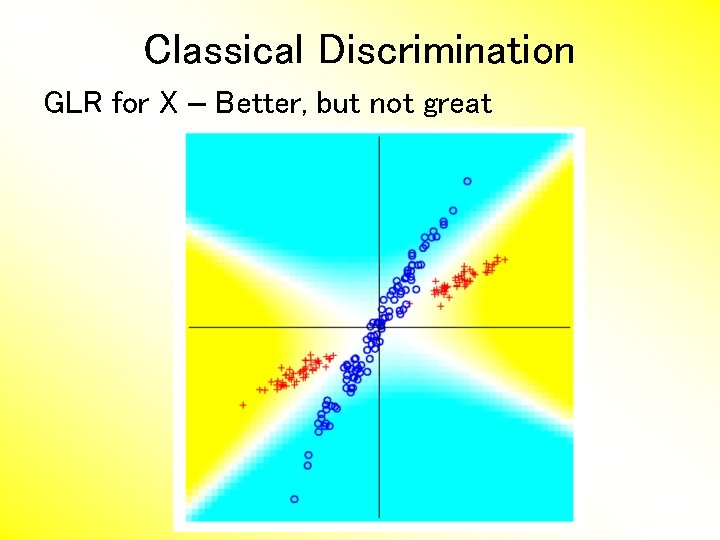Classical Discrimination GLR for X – Better, but not great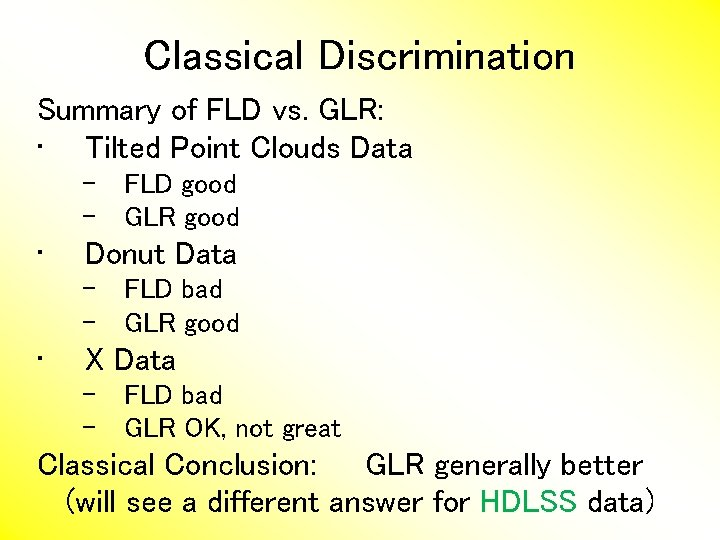Classical Discrimination Summary of FLD vs. GLR: • Tilted Point Clouds Data – FLD good – GLR good • Donut Data – FLD bad – GLR good • X Data – FLD bad – GLR OK, not great Classical Conclusion: GLR generally better (will see a different answer for HDLSS data)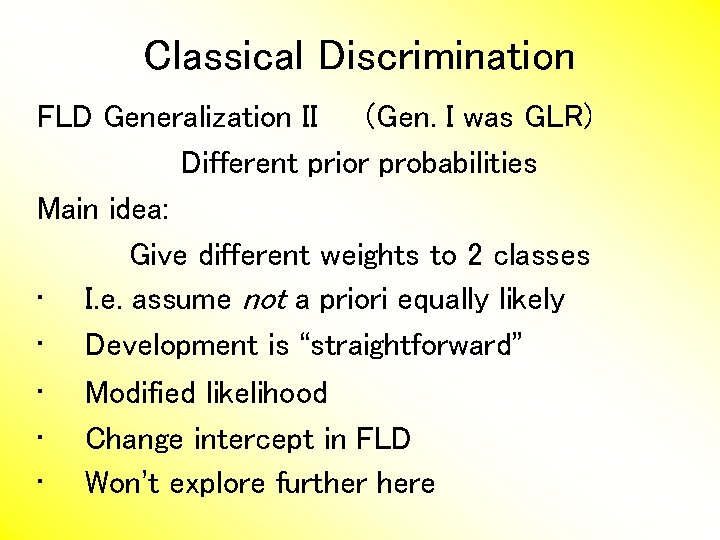Classical Discrimination FLD Generalization II (Gen. I was GLR) Different prior probabilities Main idea: Give different weights to 2 classes • I. e. assume not a priori equally likely • Development is “straightforward” • Modified likelihood • Change intercept in FLD • Won’t explore further hereClassical Discrimination •Classical Discrimination Principal Discriminant Analysis (cont. ) Simple way to find “interesting directions” among the means: PCA on set of means (Think Analog of Mean Difference)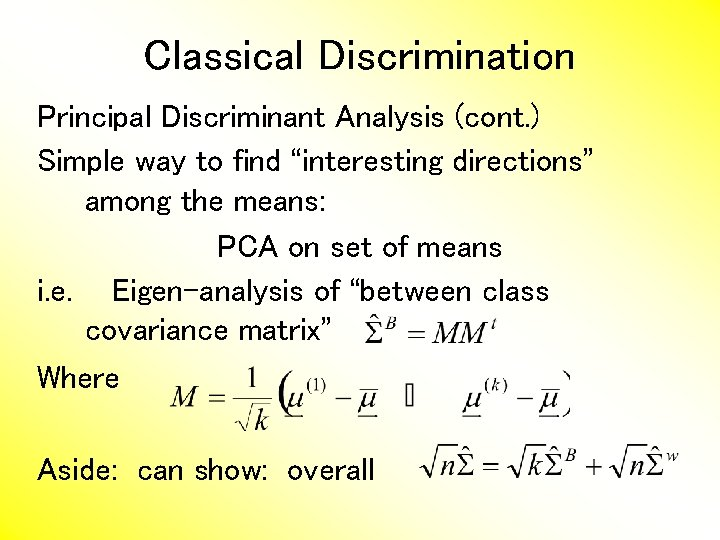Classical Discrimination Principal Discriminant Analysis (cont. ) Simple way to find “interesting directions” among the means: PCA on set of means i. e. Eigen-analysis of “between class covariance matrix” Where Aside: can show: overall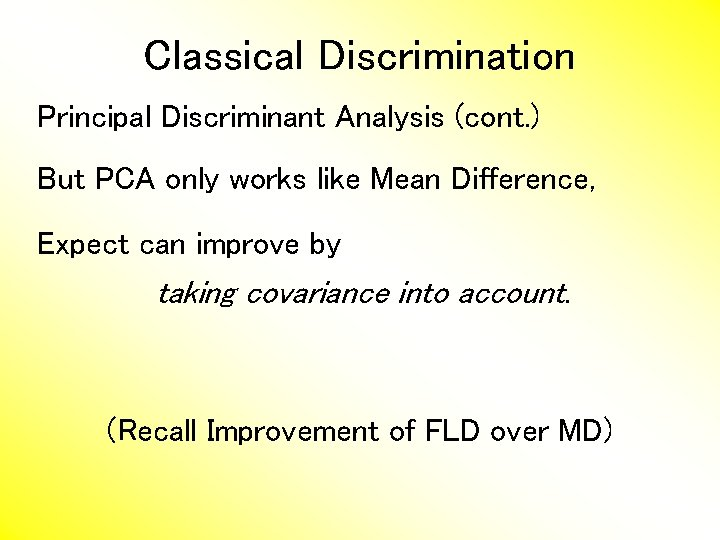Classical Discrimination Principal Discriminant Analysis (cont. ) But PCA only works like Mean Difference, Expect can improve by taking covariance into account. (Recall Improvement of FLD over MD)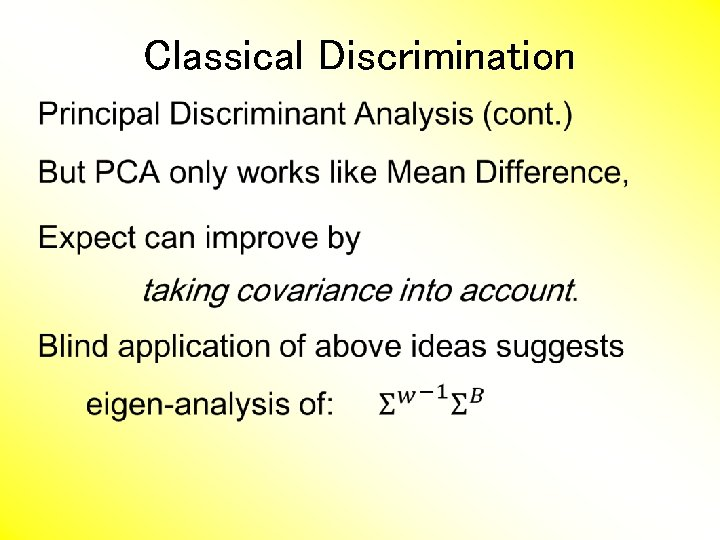Classical Discrimination •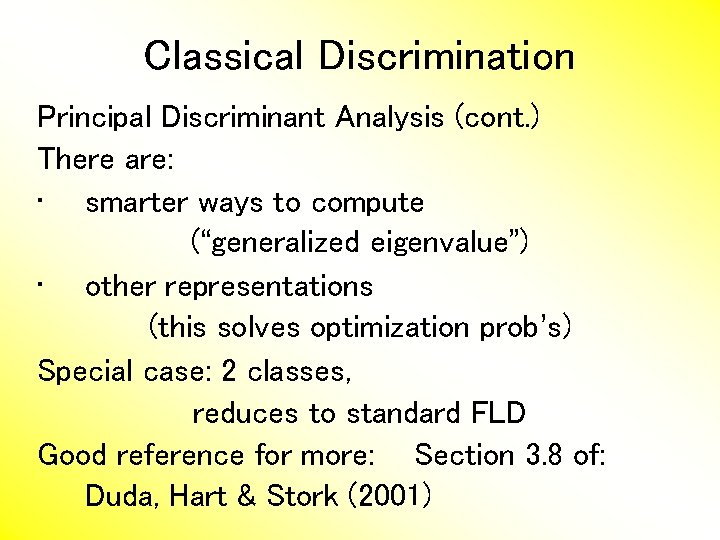Classical Discrimination Principal Discriminant Analysis (cont. ) There are: • smarter ways to compute (“generalized eigenvalue”) • other representations (this solves optimization prob’s) Special case: 2 classes, reduces to standard FLD Good reference for more: Section 3. 8 of: Duda, Hart & Stork (2001)Classical Discrimination Summary of Classical Ideas: • Among “Simple Methods” – MD and FLD sometimes similar – Sometimes FLD better – So FLD is preferred • Among Complicated Methods – GLR is best – So always use that? • Caution: – Story changes for HDLSS settings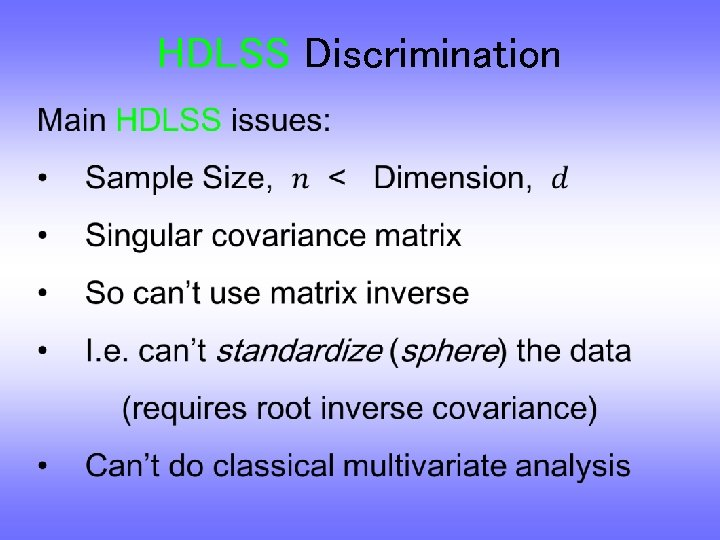HDLSS Discrimination •HDLSS Discrimination •HDLSS Discrimination An • • • approach to non-invertible covariances: Replace by generalized inverses Sometimes called pseudo inverses Note: there are several Here use Moore Penrose inverse As used by Matlab (pinv. m) Often provides useful results (but not always)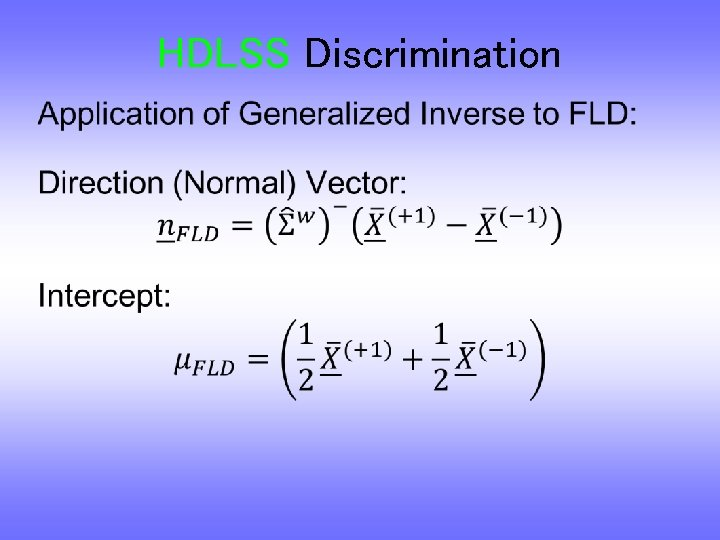HDLSS Discrimination •HDLSS Discrimination •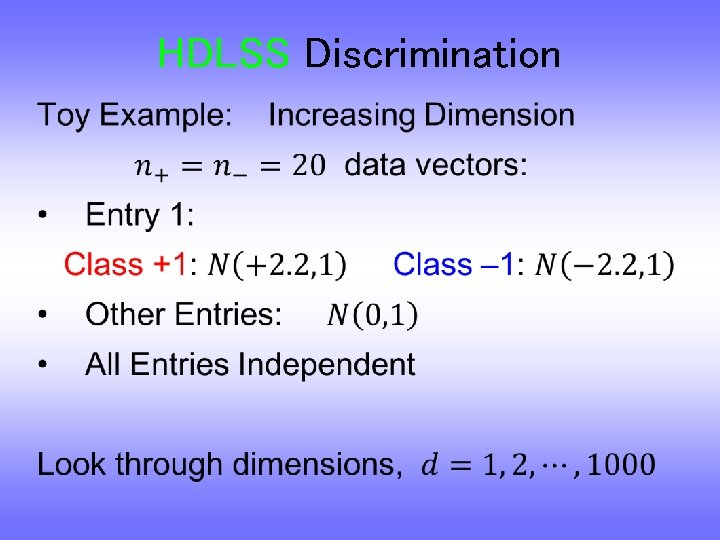HDLSS Discrimination •HDLSS Discrimination Increasing Dimension Example Project on Optimal Direction Project on FLD Direction Project on Both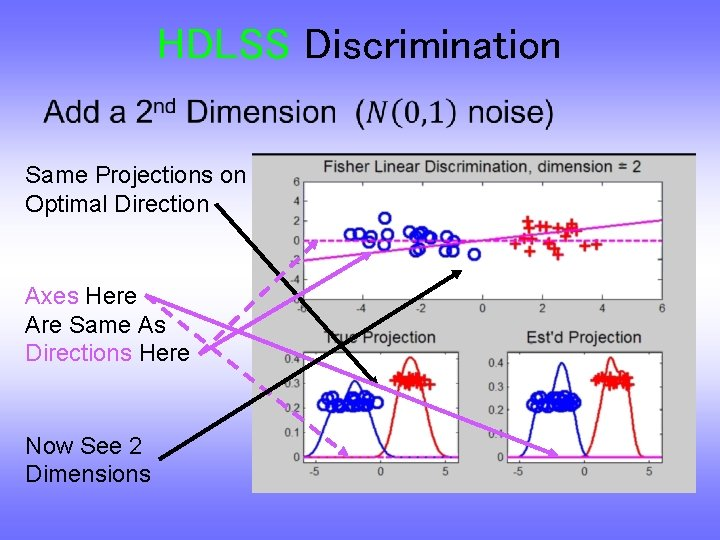HDLSS Discrimination • Same Projections on Optimal Direction Axes Here Are Same As Directions Here Now See 2 Dimensions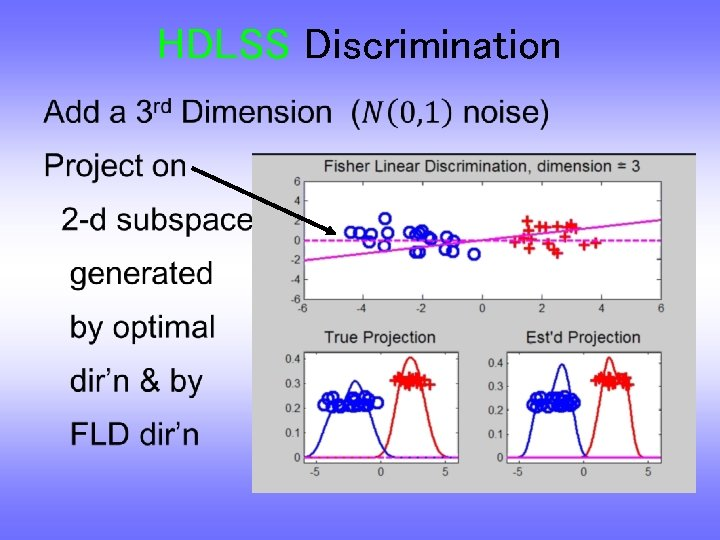HDLSS Discrimination •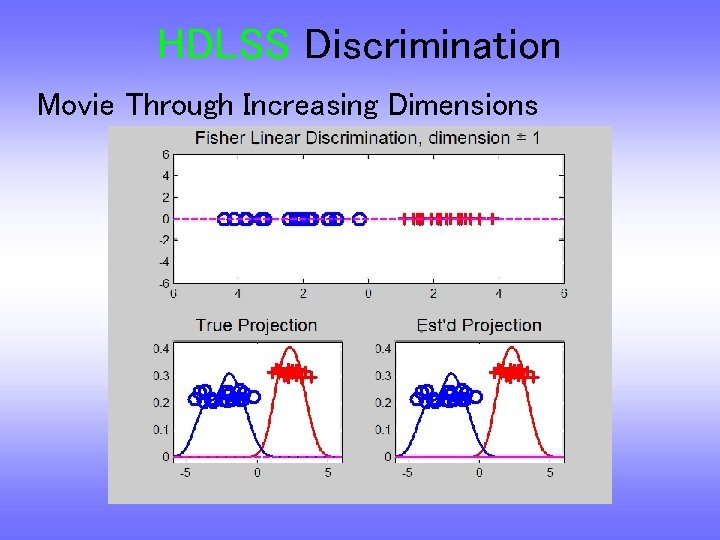HDLSS Discrimination Movie Through Increasing Dimensions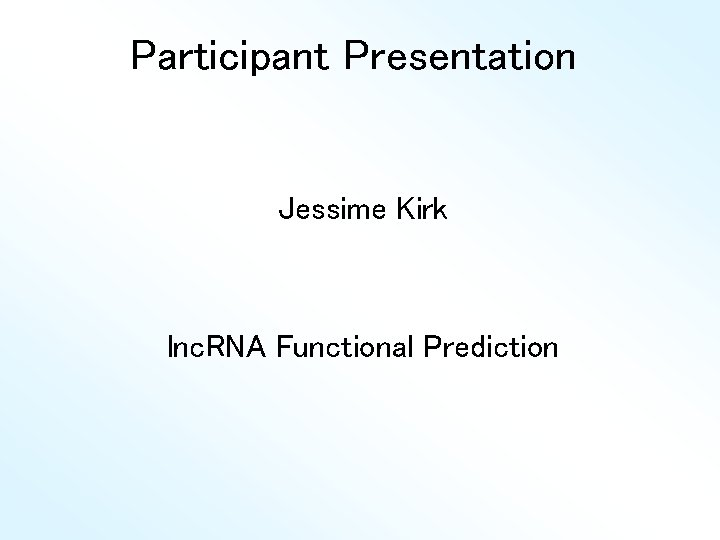Participant Presentation Jessime Kirk lnc. RNA Functional Prediction